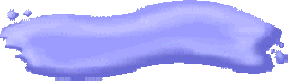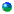History of Courses TaughtMath 105, Elementary Probability and StatisticsMath 225, Survey of Probability and StatisticsMath 244, Introduction to ProbabilityMath 279, Statistics and Probability for EngineersMath 333, Introduction to Statistics and ProbabilityMath 341, Introduction to Statistical MethodsMath 345, Introduction to Statistical MethodsMath 446, Time Series analysisMath 646, Time Series analysisMath 661, Applied StatisticsMath 662, Probability DistributionsMath 663, Introduction to BiostatisticsMath 665, Statistical InferenceMath 664, Methods for Statistical ConsultingMath 691, Stochastic Processes with ApplicationsMath 763, Generalized Linear Models